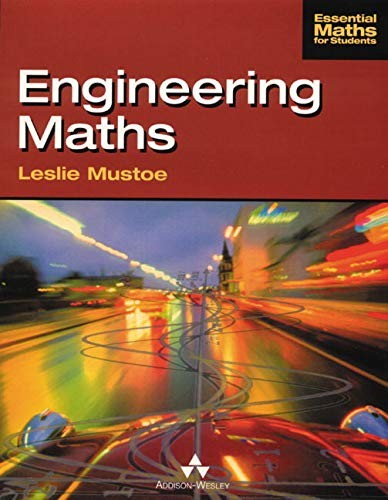# Engineering Maths by L.R. Mustoe

In Stock
£13.99
Adopts an informal, user-friendly approach, describing mathematical processes in everyday language. Mathematical ideas are then developed by example rather than by formal proof. Covers the first-year syllabus for engineering students; topics covered are widely applicable and those upon which work in subsequent years can build.
Only 2 left

## Engineering Maths Summary

### Engineering Maths by L.R. Mustoe

Engineering Maths uses an informal and user-friendly approach to provide first year engineering students with a solid mathematical base for their subsequent years of study. Essential topics are covered clearly and concisely and key ideas are highlighted and developed through detailed examples rather than formal proofs. Extensive exercises and self-assessment questions guide student understanding and help build the confidence to apply maths to the solution of engineering problems.

Ideal for first year mathematics elements of degree and diploma courses in all engineering disciplines.

### Why buy from World of BooksOur excellent value books literally don't cost the earthFree delivery in the UKEvery used book bought is one saved from landfill

1. Discrete Mathematics.
2. Sequences and Series.
3. Functions I.
4. Functions II.
5. Trigonometric and Hyperbolic Identities.
6. Differentiation I.
7. Maximum and Minimum Values.
8. Integration.
9. Applications of Integration.
10. Vectors I.
11. Vectors II.
12. Matrices and Determinants.
13. Linear Equations.
14. Iteration.
15. Complex Numbers.
16. Differentiation II.
17. Power Series.
18. Partial Differentiation.
19. Numerical Integration.
20. Ordinary Differential Equations.
21. First-Order Ordinary Differential Equations.
22. Second-Order Ordinary Differential Equations.
23. Laplace Transforms.

GOR002799704
Engineering Maths by L.R. Mustoe
L.R. Mustoe
Essential Maths For Students
Used - Very Good
Paperback
Pearson Education (US)
1997-05-21
456
0201178036
9780201178036
N/A
Book picture is for illustrative purposes only, actual binding, cover or edition may vary.
This is a used book - there is no escaping the fact it has been read by someone else and it will show signs of wear and previous use. Overall we expect it to be in very good condition, but if you are not entirely satisfied please get in touch with us.